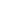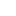# Deep Learning Baseline with TensorFlow: 10-minute setup

Those who build Deep Neural Networks often find themselves in situations when they need to develop a model that shows promising results and fast. A common reason for this is to show that meaningful prediction is possible on the available data. Another popular reason is to build the proof of concept for the project. Therefore, creating a fast baseline solves a lot of initial challenges with the ML project.

This article will show you how to build a baseline Deep Learning image recognition model using the TensorFlow framework, and more specifically, its higher-level partner Keras.

## What is a Baseline? And Why Do We Need It?

Baseline is a type of model, which serves as a benchmark of the possible capabilities on the available data. It is usually a variant of the simple basic model, which will show us the minimal possible result. Yet, it leaves a lot of room for potential further improvement. It should highlight what the most straightforward solution can achieve.

## Why TensorFlow and Keras?

### TensorFlow

TensorFlow is an open-source library and platform in Python. Its goal is to implement more convenient ways of working with Machine Learning models. More specifically, it focuses on Deep Neural Networks.

It was developed and is continuously supported by Google, so it has very high reliability. One of the most significant advantages of TensorFlow is the support of parallel computing on different GPUs. This feature made the library as popular as it is today.

While also being one of the fastest Deep Learning libraries, it supports both low-level and high-level functionalities. You can write every property of the Neural Network layer, activation function, or loss parameters for yourself or delegate the common definitions to the library. This option dramatically helps diversify the usage of Tensorflow. One of the most popular ways of using the high-level side of it is through Keras.

### Keras

Keras is also an open-source Deep Learning library in Python. It focuses on high-level commands and uses TensorFlow under the hood, so it is a top-rated Cross-Entropy tool for building Deep Learning models. According to a survey, conducted by Kaggle in October 2018, 60% of data scientists have used Keras in their work.

More importantly, it is possible to build these baselines for your specific application, as Keras includes many pre-designed and pre-trained models.

## So, How Do We Develop a Deep Learning Baseline?

Suppose you want to quickly build a model to recognize different physical objects in images. For example, you develop some horse racing predictions model and want to know if the object is a horse or a human. For this reason, we would need a simpler basic model to realize it.

Keras gives us the opportunity to use a wide range of pre-trained models for this task. We will use the classic Inception neural network in this particular case. It was pre-trained on 1000 classes from the renowned ImageNet dataset. It also has one of the highest classification accuracies among the pre-trained Keras models. We can easily change the last layer, meaning it is easy to adjust for any classification problem. Let’s take a look at how to do this.

TensorFlow and Keras are easy to install. If you haven’t done so yet, just follow the extensive guide by TensorFlow itself. This code is valid for the up-to-date versions of TF 2.4.1 and Keras 2.4.0. Once you complete the installations, you are ready for baseline development!

First, let’s load the libraries we need for our baseline:

```from keras.applications.inception_v3 import InceptionV3
from keras.preprocessing import image
from keras.models import Model, load_model, Sequential
from keras.layers import Dense, GlobalAveragePooling2D
from keras.initializers import RandomNormal
from keras.preprocessing.image import ImageDataGenerator
from keras import backend as K
from keras.callbacks import ModelCheckpoint
from keras.optimizers import SGD
import pathlib
from tensorflow.keras import utils
from tensorflow.keras.preprocessing import image_dataset_from_directory
from tensorflow.keras.layers.experimental.preprocessing import
Rescaling
import tensorflow_datasets as tfds```

We will use the tf-flowers dataset for this project. It is one of the inbuilt datasets used for image recognition with TensorFlow. Therefore, we can load and process it using standard functions. The dataset contains 3 670 images of five flower types: daisies, dandelions, roses, sunflowers, and tulips.

```data_url = "https://storage.googleapis.com/download.tensorflow.org/”
example_images/flower_photos.tgz"
data_dir = utils.get_file(
origin=data_url,
fname='flower_photos',
untar=True
)
data_dir = pathlib.Path(data_dir)

```

Then, we need to preprocess the images to have the format applicable for our Inception network and split the dataset into training and validation:

```batch_size = 32
img_height = 299
img_width = 299

train_ds = image_dataset_from_directory(
data_dir,
validation_split=0.2,
subset="training",
seed=123,
image_size=(img_height, img_width),
batch_size=batch_size
)

val_ds = image_dataset_from_directory(
data_dir,
validation_split=0.2,
subset="validation",
seed=123,
image_size=(img_height, img_width),
batch_size=batch_size
)

normalization_layer = Rescaling(1./255)

train_ds = train_ds.map(lambda x, y: (normalization_layer(x), y))
val_ds = val_ds.map(lambda x, y: (normalization_layer(x), y))```

Now it’s time to load our pre-trained Inception model. We can easily do so with one expression:

```base_model = InceptionV3(
weights='imagenet',
include_top=False,
input_shape=(299, 299, 3),
classes=5,
classifier_activation="softmax"
)
```

We need to attach the classifying layer to the model to serve our purposes. In this case, the number of output classes corresponds to the five classes of the tf-flowers dataset. Another point to make is the input image shape.  For any dataset, the shape corresponds to the image size, which is 32×32 in our case. We use the channel-last approach, so the number of our RGB channels goes last.

Probably the most important line here is the “weights=’imagenet’”. This parameter indicates that we will be using weights pre-trained by Google on the ImageNet dataset. The apparent advantage is a chance to receive satisfying results even if we train only the last layers.

```base_model.trainable = False

model = Sequential([
base_model,
GlobalAveragePooling2D(),
Dense(10, activation='softmax', kernel_initializer=RandomNormal())
])

```

By running the summary of the model, we can review its structure:

```base_model.summary()

model.summary()
```

So, we created the model with slightly more than 21,8 million parameters, with only 10,000 of them having the possibility to change. These conditions allow us to train the network in a reasonable time frame, even in a non-advanced setup. Since we want to use all the advantages of ImageNet pre-training and show the power of the raw baseline, we will freeze all the original layers. Freezing will block them from changes and speed up the model training, relying on pre-trained specifics.

```for layer in model.layers:
print(layer.trainable)
```

This option will work well enough only if the classes on your and the original data are similar. Then the network will still have enough pre-learned features to recognize your objects. However, the variety of 1000 classes in the ImageNet dataset means it works for many applications.

In other cases, when the objects you want to detect significantly differ from the original classes, you would probably want to unfreeze some more layers. The best possible way to do this is to unfreeze some of the top convolutional layers. These layers will be responsible for picking up the smallest details of the images to allow the network to better adjust to their features.

The data is ready so it’s time to compile and train our model! We will train it for the standard 30 epochs. We will also use the classic stochastic gradient descent (SGD) method to show the possible baseline results. The classification accuracy will serve as our metric and Categorical Cross-Entropy as the loss. Both are standard methods for Keras.

```model.compile(
optimizer=SGD(learning_rate=0.0005, momentum=0.9),
loss='categorical_crossentropy',
metrics=['accuracy']
)

model.fit(
train_ds,
epochs=30,
verbose=1,
validation_data=val_ds
)
```

As we can see, our performance is pretty impressive even with the standard settings of the frozen pre-trained model. The resulting validation accuracy is 89,92%, which is an impressive starting point for the 5-class classification problem.

However, let’s try to unfreeze the last convolutional layers of the loaded Inception model until the previous concatenation layer and examine how it changes the performance. This also makes just over 6 million parameters in the model training, while another 15,7 million in the base model starting layers remain frozen.

```base_model.trainable = True
for layer in base_model.layers[:-32]:
layer.trainable = False
```

Even though the time of training significantly increased, so did our test accuracy!

It outperformed our initial baseline model after the 4th iteration of learning, showing a validation accuracy of 90,46%. Still, overfitting with the same hyperparameters started around this point, so additional tuning is a good idea for further learning. The unfrozen model, however, did reach a validation accuracy of 91,42%.

Let’s look at how it predicts several images in the baseline variant.Picture 1. Correctly classified images from tf-flowers dataset with the baseline model

And now let’s see the examples of image classes the model got wrong.Picture 2. Examples of incorrectly classified images from the tf-flowers dataset with the baseline model

The model achieved this performance level without any hyperparameter tuning or method choosing techniques with the standard settings and the most classic methods. Therefore, it does what a baseline needs to do first and foremost: show usable performance while leaving room for development.

## Conclusions

The baseline models are needed to quickly show possible results while doing so with the closest tools available. It makes sense to develop such a model before any serious analysis. Afterward, it can be improved or changed if needed.

In any case, your baseline should indicate how good the data is for the given problem or how well it is performing with the general approaches. It will also show what minimum results you can expect from further model improvement.(2 votes, average: 4.00 out of 5)Loading...